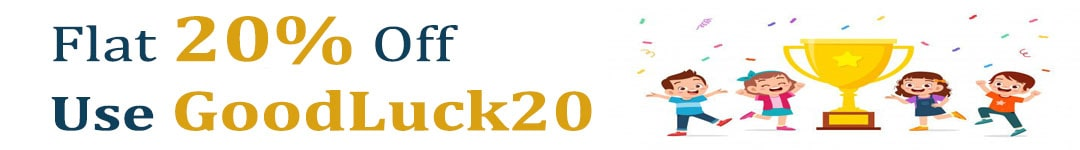International Maths Olympiad Forum By SOF Olympiad Trainer - Page 627

# User ForumSubject :IMO    Class : Class 3

## Ans 1:

Class : Class 6
C is the right answer. TV costs 2930 and PS costs 1870 less than 2930 i.e. PS costs 1060 Hence total of 2930 and 1060 is the answer

## Ans 2:

Class : Class 5
c is correct

Subject :IMO    Class : Class 6

#### View All Answers (0)

Subject :IMO    Class : Class 6

## Ans 1:

Class : Class 8
Plz explain ?????????????

Class : Class 3
kindly explain

Class : Class 9
16 is the answer

## Ans 4:

Class : Class 6
plz provide the solution for the same.

Class : Class 8

Class : Class 6

## Ans 7:

Class : Class 9
Please provide the solution

## Ans 8:

Class : Class 8
i guess a is 8 ,b is 4, c is 4

Subject :IMO    Class : Class 6

Class : Class 6
C3

## Ans 2:

Class : Class 7
X,t,a and b have 1line symmetry. So and should be D4

## Ans 3:

Class : Class 6
only 3 a b and t have only one symmetry line

## Ans 4:

Class : Class 6

Subject :IMO    Class : Class 5

Class : Class 7

## Ans 2:

Class : Class 6

Subject :IMO    Class : Class 4

Class : Class 4
51

## Ans 2:

Class : Class 8
Ans:B 51 As 9+11=20, 11+20=31, 20+31=51, 31+51=82

Subject :IMO    Class : Class 6

## Ans 1:

Class : Class 8
the solution is not clear

## Ans 2:

Class : Class 8
5 years ago trishikas age is 10 yrs jay is 3x*10=30x present age of trishika is 15 yrs and jay is 30x +5 after 3 yrs trishika is 18 and jay is 30x+8 total age = 30x+8+18=30x+26 Pravir

Class : Class 6

## Ans 4:

Class : Class 7
I didn't understand the solution completely

## Ans 5:

Class : Class 4
5 years ago, Trishika was 10 yrs old. Then, Jay was 3 x (10) yrs old = 30x 3 years after means 8 years after. Trishikaâs age = 10 8 = 18 yrs. Jayâs age = (30x) 8 yrs. Total = 18 (30x) 8 = 26 30x Answer-(D) (26 30x) years old

## Ans 6:

Class : Class 8
5 years ago trishikas age is 10 yrs jay is 3x*10=30x present age of trishika is 15 yrs and jay is 30x +5 after 3 yrs trishika is 18 and jay is 30x+8 total age = 30x+8+18=30x+26 Pravir

Subject :IMO    Class : Class 3

Class : Class 4

Class : Class 4

## Ans 3:

Class : Class 8
option D should be right answer

## Ans 4:

Class : Class 3
A.quarter of 16 is 4 and 3/4 of that is 3,so answer is A,3

## Ans 5:

Class : Class 3
correct answer should be D

Class : Class 3

## Ans 7:

Class : Class 3
ans is a or explain

## Ans 8:

Class : Class 7
3/4th of quater is 3/4 x 1/4 = 3/16, therefore the answer is A

## Ans 9:

Class : Class 6
Answer is A because the questions asks 3/4 of Quarter of traiangle i.e. 1/4th. So it is basically asking 3/16 of the triangle, hence, A.

## Ans 10:

Class : Class 4
answer is D A. 3/16 which is in lowest form B.4/16 =1/4 C.2/16=1/8 D.12/16=3/4

Subject :IMO    Class : Class 5

## Ans 1:

Class : Class 8
Whatâs the correct answer?

## Ans 2:

Class : Class 6
The answer will be C, Saksham.

Subject :IMO    Class : Class 3

## Ans 1:

Class : Class 3
this quetion is wrong

## Ans 2: (Master Answer)

Class : Class 1
The correct answer is C.